# Make an RGB cube with Python and Scribus

## Create a 3D cube that shows the RGB color spectrum with Scribus' Python Scripter capability.Image by :
Opensource.com

## Subscribe now

Get the highlights in your inbox every week.

When I decided I wanted to play with color this summer, I thought about the fact that colors are usually depicted on a color wheel. This is usually with pigment colors rather than light, and you lose any sense of the variation in color brightness or luminosity.

As an alternative to the color wheel, I came up with the idea of displaying the RGB spectrum on the surfaces of a cube using a series of graphs. RGB values would be depicted on a three-dimensional graph with X-, Y-, and Z-axes. For example, a surface would keep B (or blue) at 0 and the remaining axes would show what happens as I plot values as colors for R (red) and G (green) from 0 to 255.

It turns out this is not very difficult to do using Scribus and its Python Scripter capability. I can create RGB colors, make rectangles showing the colors, and arrange them in a 2D format. I decided to make value jumps of 5 for the colors and make rectangles measuring 5 points on a side. Thus, for each 2D graph, I would make about 250 colors, and the cube would measure 250 points to a side, or 3.5 inches.

I used this bit of Python code to accomplish that task for the Green–Red graph:

```x = 300
y = 300
r = 0
g = 0
b = 0

if scribus.newDoc(scribus.PAPER_LETTER, (0,0,0,0),scribus.PORTRAIT, 1,                  scribus.UNIT_POINTS, scribus.NOFACINGPAGES, scribus.FIRSTPAGERIGHT):
while r < 256:
while g < 256:
newcolor = str(r) + '_' + str(g) + '_' + str(b)
if newcolor == '0_0_0':
newcolor = 'Black'
scribus.defineColorRGB(newcolor,r, g, b)
rect = scribus.createRect(x + g, y, 5, 5)
scribus.setFillColor(newcolor, rect)
scribus.setLineColor(newcolor, rect)
g = g + 5
g = 0
r = r + 5
y = y – 5```

This script starts the graphical structure at 300, 300, which is about the middle of a US Letter-size page horizontally and maybe a third of the way down from the top; this is the origin of the graph. Then it builds the graph horizontally along the X-axis (the Green value), then returns to the Y-axis, jumps up the page 5 points, and makes another line of rectangles.

## redgreengraph.png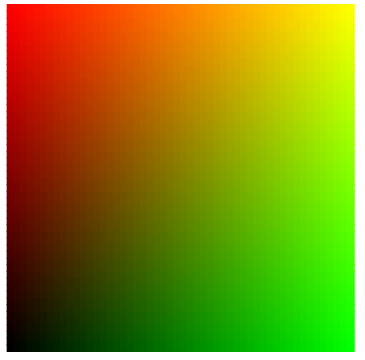That looks easy enough; I'll just fiddle with the numbers and make the other sides. But this isn't just a matter of making two more graphs, one with Blue–Green and another with Red–Blue. I had in mind to create an unfolded cube so I could print it, cut it, fold it, and create a 3D view of RGB. Therefore, the next part (going down the page) needs to have the origin (the Black corner) at the upper left, with Green horizontally and Blue vertically increasing downward.

"Fiddling with the numbers" ended up being more or less trial and error to get what I wanted. After creating the second graph, I needed the third one, for Red–Blue, to have the origin in the upper left corner with Red increasing to the left and Blue increasing downward.

Here it is:

## rgbcubefirsthalf.png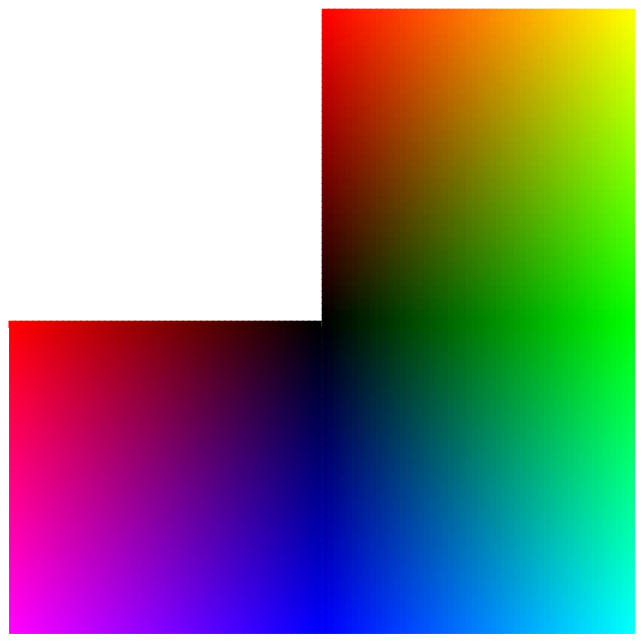Of course, this is just the first half of this cube. I needed to make a similar shape, except that the origins should be White (rather than Black) to represent the high values. It's one of those times when I wish I were smarter, since not only did I need to make a similar overall shape, it needed to interface with the first shape in a mirror-image sort of way (I think). Sometimes trial and error is the only friend you have.

Here is how that came out; I used a separate script since there wasn't enough space on a US Letter-sized page for both of them:

## rgbcubesecondhalf.png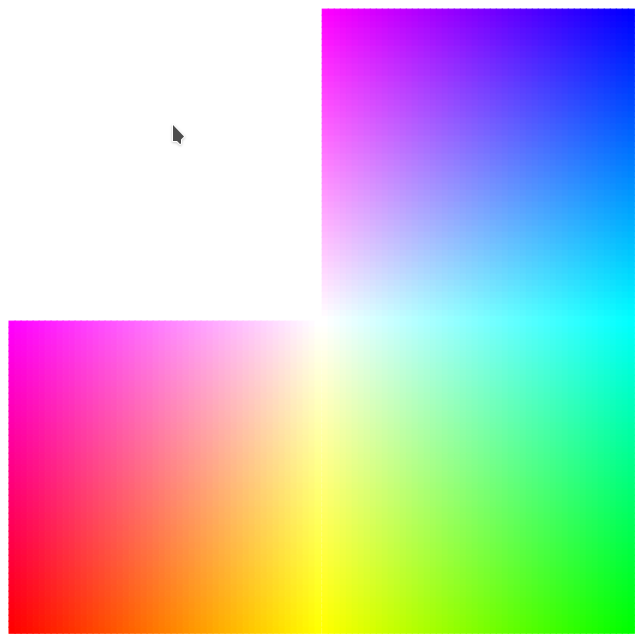Now, it's off to the printer! This is where you get a sense of how well your color printer does with RGB to CMYK transformation as well as other aspects of printing color-dense spaces.

Next, boys and girls, it's cut-and-paste time! I could use tape, but I didn't want to change the appearance of the surfaces, so I left some tabs along the sides while cutting so I could glue them on the inside. From experience, I can say that printing on copy paper comes out with some undesirable wrinkles, so after my copy paper prototype, I printed the cube on heavier paper with a matte finish.

## rgbcubecompositephoto.png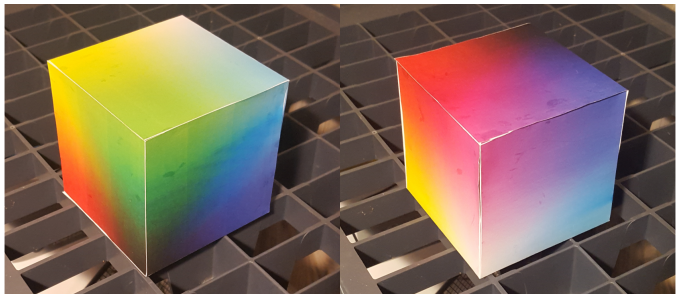Keep in mind this is just a view of the boundaries of the RGB space; to be more accurate, you would have to make a solid cube that you could slice in the middle. For example, this would be a slice through a solid RGB cube where Blue = 120:

## rgbcubeslice.png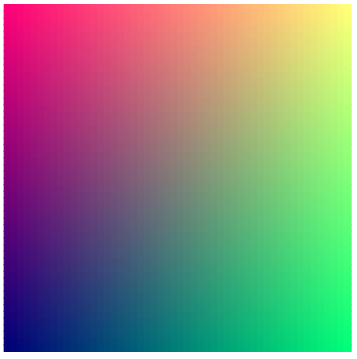In the end, I had fun doing this project. In case you want to join the party, here are the two scripts. These scripts require Scribus 1.5.x, which provides the defineColorRGB() function.

Here's the first half:

```#!/usr/bin/env python
# black2rgb.py
"""
Creates one-half of RGB cube with Black at origin
"""

import scribus

x = 300
y = 300
r = 0
g = 0
b = 0

if scribus.newDoc(scribus.PAPER_LETTER, (0,0,0,0),scribus.PORTRAIT, 1, scribus.UNIT_POINTS, scribus.NOFACINGPAGES, scribus.FIRSTPAGERIGHT):
while r < 256:
while g < 256:
newcolor = str(r) + '_' + str(g) + '_' + str(b)
if newcolor == '0_0_0':
newcolor = 'Black'
scribus.defineColorRGB(newcolor,r, g, b)
rect = scribus.createRect(x + g, y, 5, 5)
scribus.setFillColor(newcolor, rect)
scribus.setLineColor(newcolor, rect)
g = g + 5
g = 0
r = r + 5
y = y - 5

r = 0
g = 0
y = 305

while b < 256:
while g < 256:
newcolor = str(r) + '_' + str(g) + '_' + str(b)
if newcolor == '0_0_0':
newcolor = 'Black'
scribus.defineColorRGB(newcolor,r, g, b)
rect = scribus.createRect(x + g, y, 5, 5)
scribus.setFillColor(newcolor, rect)
scribus.setLineColor(newcolor, rect)
g = g + 5
g = 0
b = b + 5
y = y + 5

r = 255
g = 0
y = 305
x = 39
b = 0

while b < 256:
while r >= 0:
newcolor = str(r) + '_' + str(g) + '_' + str(b)
if newcolor == '0_0_0':
newcolor = 'Black'
scribus.defineColorRGB(newcolor,r, g, b)
rect = scribus.createRect(x, y, 5, 5)
scribus.setFillColor(newcolor, rect)
scribus.setLineColor(newcolor, rect)
r = r - 5
x = x+5
b = b + 5
x = 39.5
r = 255
y = y + 5

scribus.setRedraw(True)
scribus.redrawAll()```

Now the second half:

```#!/usr/bin/env python
# white2rgb.py
"""
Creates one-half of RGB cube with White at origin
"""

import scribus

x = 300
y = 300
r = 255
g = 255
b = 255

if scribus.newDoc(scribus.PAPER_LETTER, (0,0,0,0),scribus.PORTRAIT, 1, scribus.UNIT_POINTS, scribus.NOFACINGPAGES, scribus.FIRSTPAGERIGHT):
while g >= 0:
while r >= 0:
newcolor = str(r) + '_' + str(g) + '_' + str(b)
if newcolor == '255_255_255':
newcolor = 'White'
scribus.defineColorRGB(newcolor,r, g, b)
rect = scribus.createRect(x + 255 - r, y, 5, 5)
scribus.setFillColor(newcolor, rect)
scribus.setLineColor(newcolor, rect)
r = r - 5
r = 255
g = g - 5
y = y - 5

r = 255
g = 255
y = 305

while b >= 0:
while r >= 0:
newcolor = str(r) + '_' + str(g) + '_' + str(b)
if newcolor == '255_255_255':
newcolor = 'White'
scribus.defineColorRGB(newcolor,r, g, b)
rect = scribus.createRect(x + 255 - r, y, 5, 5)
scribus.setFillColor(newcolor, rect)
scribus.setLineColor(newcolor, rect)
r = r - 5
r = 255
b = b - 5
y = y + 5

r = 255
g = 0
y = 305
x = 39
b = 255

while b >= 0:
while g < 256:
newcolor = str(r) + '_' + str(g) + '_' + str(b)
if newcolor == '255_255_255':
newcolor = 'White'
scribus.defineColorRGB(newcolor,r, g, b)
rect = scribus.createRect(x + g, y, 5, 5)
scribus.setFillColor(newcolor, rect)
scribus.setLineColor(newcolor, rect)
g = g + 5
g = 0
b = b - 5
y = y + 5

scribus.setRedraw(True)
scribus.redrawAll()```

Since I was creating a large number of colors, I wasn't surprised to see that the Scribus file is much larger than the PDF I made from it. For example, my Scribus SLA file was 3.0MB, while the PDF I generated from it was only 70KB.